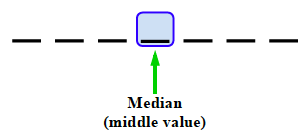### Home > CC2MN > Chapter 1 > Lesson 1.1.4 > Problem1-35

1-35.

Read the Math Notes box for this lesson and review the information about how to find the median of a data set. Then find the median for Andy’s test scores: $76$, $84$, $93$, $67$, $82$, $87$, and $76$.

The median is the middle number in a set of data arranged numerically.To find the middle value, put the data in order from least to greatest.